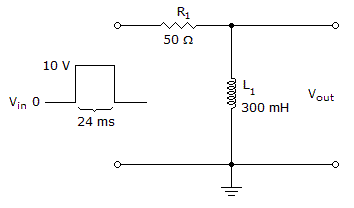# Electronics - Time Response of Reactive Circuits - Discussion

### Discussion :: Time Response of Reactive Circuits - General Questions (Q.No.12)

12.What is the voltage across the inductor in the given circuit on the falling edge of the first input pulse?

 [A]. –0.2 V [B]. 0.2 V [C]. –9.8 V [D]. 9.8 V

Explanation:

No answer description available for this question.

 Vasu said: (Oct 14, 2011) Can anyone explain this please ?

 Muddassir said: (Jun 24, 2012) Toff= RL=RC. Ton is given. T=Toff+Ton. Ton/T=V (voltage across the inductor). Put the Value & get the result.

 Ramesh said: (May 8, 2015) Voltage across inductor VL = vin(1-e^-Rt/L). R = 50 ohms, L= 300 mH, t = 24 ms. Vr = VL-Vin.

 Meenu said: (Oct 20, 2015) Toff = L/R = 6 ms. Ton = 24 ms. T = Toff+Ton = 24 ms+6 ms = 30 ms. VL = Ton/T = 24 ms/30 ms = 0.8. Vf = Vl-Vin = 0.8-10.0 = -0.2 ms.

 Pooja said: (Mar 15, 2016) @Meenu 0.8-10.0 = -0.2?

 Retsam said: (Jul 27, 2018) Why in negative?

 Marie said: (Mar 10, 2019) VR = Vin(1-(e^(-Rt/L)). VR = 10(1-(e^(-((24x10^(-3))(50))/(300x10^-3)), VR = 9.8V, VL = VR - VIN. VL = 9.8-10 = -0.2V.